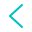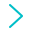# Digital LCD thermometer – Learning path project 14Our Learning Path is an itinerary designed to teach all the foundaments and a little more about electronics, coding and robotics.

When you complete all the projects you will be able to develop almost any project you can imagine. In each chapter new concepts are introduced. You will learn by doing in a funny way.

Learn how to measure ambient temperature and show it in your LCD display building this fantastic thermometer

DIGITAL THERMOMETER WITH LCD DISPLAY
Project 14

Combine sensor and LCD display and refresh your learnings!

14 - Components

You already used the temperature sensor before. Our board adapts resistance to an output voltage, and works fine from -40 to 120º. Exists many resistance thermometer (RTD) according application.

Also used before, the LDC display is perfect to show many values and messages to users, combining numbers and letters.

RGB led is perfect to show clearly to user some situation emitting different colours.

14 - LCD thermometer circuit

You used all components before, so feel free to connect it now and then check below if all is fine.

Connect wires from 4in1 board to components:

- A5 S pin (blue) to SCL pin from LCD display
- A4 S pin (blue) to SDA pin from LCD display
- A4 V pin (red) to VCC pin from LCD display
- A4 G pin (black) to GND pin from LCD display

- DIO4 to RGB LED module
- A2 to Temperature sensor

14.1 - Reading temperature in Celsius and Farenheit

Here you found the conversion between analog reading and measured values.

Arduino Coding
```void setup() {
Serial.begin(9600);       // INICIALIZAR LA CONEXIÓN SERIAL

}

void loop() {

float value = analogRead(0);
float voltage= (value * 5.0 )/1024.0; // CALCULAMOS VOLTAGE
float celsius= (voltage - 0.5)*100;   //PASAMOS EL VOLTAGE A GRADOS CENTIGRADOS

float fahrenheit= (celsius * 9/5)+32;   // CONVERTIMOS LOS GRADOS CENTIGRADOS EN FAHRENHEIT
Serial.print(celsius);                  // MOSTRAMOS POR EL MONITOR SERIE LA TEMPERATURA EN CELSISUS DURANTE 1 S
Serial.print(" C \t");
delay(1000);
Serial.print("\t");
Serial.print(fahrenheit);               // MOSTRAMOS POR EL MONITOR SERIE LA TEMPERATURA EN FAHRENHEIT DURANTE 1 S
Serial.println(" F");
delay(1000);

}```

Probably your reading will be unstable, to solve it, we make several readings and we are left with an average value. Also if the measured value doesn't match with other thermometer, you need to add a calibrating variable to your temperature sensor. Try it!!

14.2 - Show temperature on display

Add the display feature see your ambient temperature. We can show measurement in celsius and farenheit degrees at same time. Remember in mBlock LCD not works in live mode. See how to add libaries if you need help.

Arduino Coding
```#include <Wire.h>
#include <LiquidCrystal_I2C.h>        // LIBRERIA NECESARIA PARA LA PANTALLA LCD

LiquidCrystal_I2C lcd(0x27,16,2);     // CONFIGURACIÓN DE LA PANTALLA LCD

void setup() {

lcd.begin();              // INICIALIZACION PANTALLA LCD
lcd.clear();              // LIMPIAR LA PANTALLA LCD

}

void loop() {

int value = analogRead(0);
float voltage= (value * 5.0 )/1024.0;       // CALCULAMOS VOLTAGE
float celsius= (voltage - 0.5)*100;         // PASAMOS EL VOLTAGE A GRADOS CENTIGRADOS
float fahrenheit= (celsius * 9/5)+32;       // CALCULAMOS LA TEMPERATURA EN FAHRENHEIT

lcd.setCursor(0,0);         // COLOCAMOS LA PRIMERA LETRA EN LA POSICIÓN (0,0) DEL DISPLAY, ES DECIR AL PRINCIPIO
lcd.print("Temp:");
lcd.setCursor(6,0);         // COLOCAMOS LOS VALORES DE LA TEMPERATURA EN LA PRIMERA FILA (FILA 0) Y COLUMNA 6
lcd.print(celsius);
lcd.setCursor(12,0);
lcd.print(" C");            // COLOCAMOS UNA "C" EN LA FILA 0 Y COLUMNA 12 PARA INDICAR QUE HABLAMOS DE CELSIUS

lcd.setCursor(6,1);
lcd.print(fahrenheit);     // COLOCAMOS LOS VALORES DE LA TEMPERATURA EN FARENHEIT LA SEGUNDA FILA (FILA 1) Y COLUMNA 6
lcd.setCursor(12,1);       // COLOCAMOS UNA "F" EN LA FILA 1 Y COLUMNA 12 PARA INDICAR QUE HABLAMOS DE FAHRENHEIT
lcd.print(" F");

delay(1000);              // ESPERAR 1 SEGUNDO HASTA MOSTRAR LOS SIGUIENTES VALORES

}```

Maybe you can add a button to change degrees Celsius to Fahrenheit in first line, and use second line for other messages. Cool!! Isn't it?

Final project - LCD Thermometer clock

Now is the time to build a complete Digital thermometer clock with LCD display. Find here cardboard template .

An RGB led shines according temperature, similar to this map. You can see our activity colour thermometer designed for the Maker Kit 2 to get a more accurate colour in your LED.

Arduino code
```#include "LiquidCrystal_I2C.h"
#include "NeoPixel.h"

float average = 0;
float voltage = 0;
float celsius = 0;
float fahrenheit = 0;
float hour = 0;
float minute = 0;
float seconds = 0;
float i = 0;

LiquidCrystal_I2C lcd_i2c_0x27(0x27, 16, 2);
Adafruit_NeoPixel strip = Adafruit_NeoPixel(1, 9, NEO_GRB + NEO_KHZ800);

void _delay(float seconds) {
long endTime = millis() + seconds * 1000;
while(millis() < endTime) _loop();
}

void setup() {
lcd_i2c_0x27.init();
lcd_i2c_0x27.backlight();
strip.begin();
pinMode(A0+2,INPUT);
pinMode(4,INPUT);

lcd_i2c_0x27.clear();

average = 0;
hour = 0;
minute = 0;
seconds = 0;
}

void loop() {
// When seconds higher than 60, update minutes. In manual adjust it could be a higher value before stay here again
if(seconds < 61){
seconds += 1;
average = 0;
// 9 passes to reduce fluctuation in sensor readings
for(int count=0;count<9;count++){
average = (average + analogRead(A0+2));
// Most cycle time here, so waiting for button pushes for clock setting
if(digitalRead(4)){
minute += 1;
}
// Delay time adjustet to better clock accuracy.
_delay(0.108);
}
average = average / 9;
// Average value from last reading and current reading to reduce fluctuations
voltage = ((voltage + (average * 5) / 1024)) / 2;
celsius = ((celsius + ((voltage - 0.5)) * 100)) / 2;
fahrenheit = (celsius * (9 / 5) + 32);
average = 0;
}
if(seconds == 60.000000){
minute += 1;
seconds = 0;
}
if(minute > 59){
hour += 1;
minute = 0;
}
if(hour == 24.000000){
minute = 0;
}
// Join al LCD update values to clear and show with minimum delay.
lcd_i2c_0x27.clear();
lcd_i2c_0x27.setCursor(2 - 1, 2 - 1);
lcd_i2c_0x27.print(String(celsius) + String("C"));
lcd_i2c_0x27.setCursor(11 - 1, 2 - 1);
lcd_i2c_0x27.print(String(fahrenheit) + String("F"));
lcd_i2c_0x27.setCursor(1 - 1, 1 - 1);
lcd_i2c_0x27.print(String(round(hour)) + String("h"));
lcd_i2c_0x27.setCursor(5 - 1, 1 - 1);
lcd_i2c_0x27.print(String(round(minute)) + String("m"));
lcd_i2c_0x27.setCursor(9 - 1, 1 - 1);
lcd_i2c_0x27.print(String(round(seconds)) + String("s"));
// RGB led color output in fuctin of temp value, cold, fine, hot.
if(celsius < 22){
strip.setPixelColor(0, 0, 0, 255);
strip.show();
}else{
if(celsius < 28){
strip.setPixelColor(0, 0, 255, 0);
strip.show();
}else{
strip.setPixelColor(0, 255, 0, 0);
strip.show();
}
}
}```The Mega Maker Kit fits perfectly with the Learning Path, you can build all projects with it, but if you have other kits, you can also follow the entire itinerary and finish some projects, or buy the missing components. You can check in our Learning Path page.

0 0

0